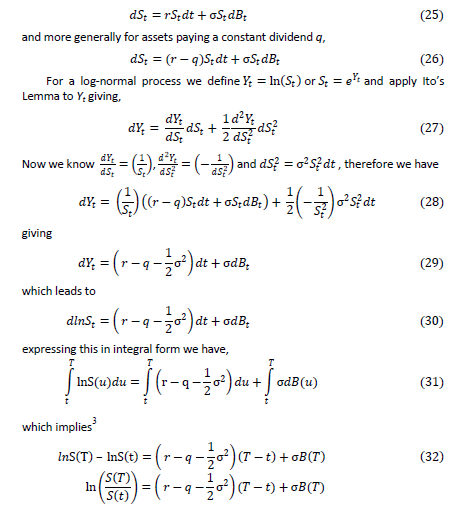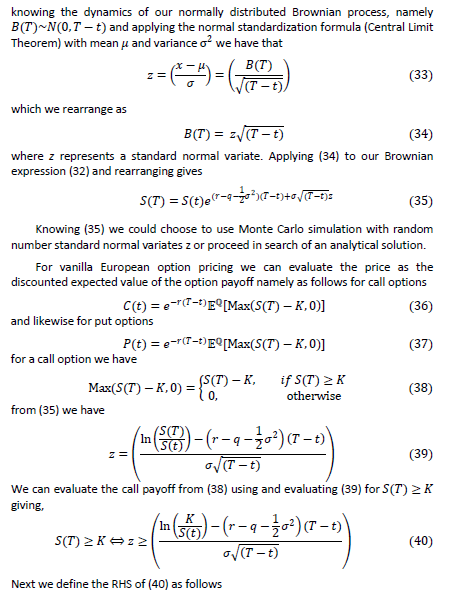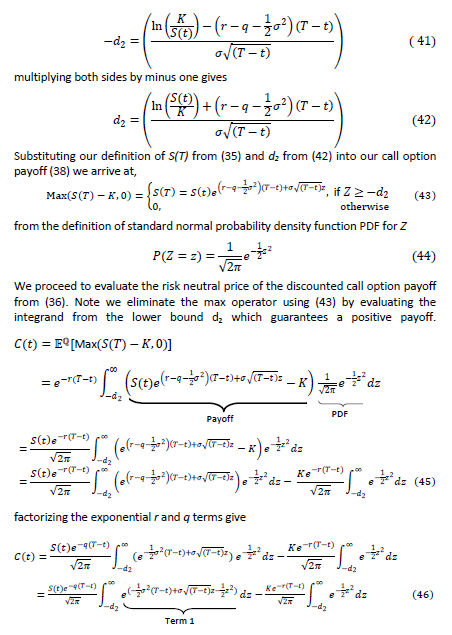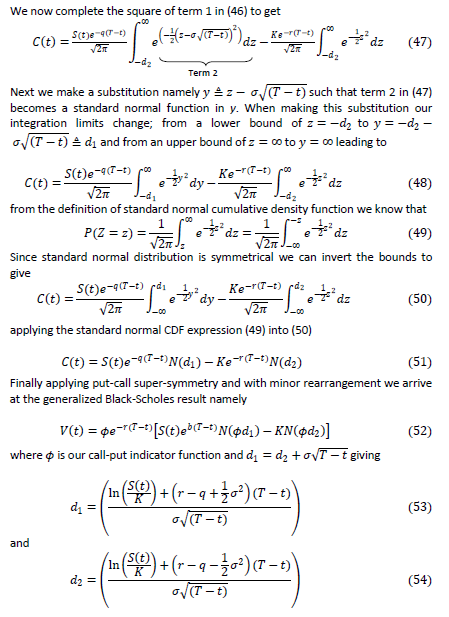Interest Rate Swaptions: A Review and Derivation of Swaption Pricing Formulae

Journal of Economics and Financial Analysis, Vol:2, No:2 (2018) 87-103

# Interest Rate Swaptions: A Review and Derivation of Swaption Pricing Formulae

Nicholas BURGESS*

## Abstract

In this paper we outline the European interest rate swaption pricing formula from first principles using the Martingale Representation Theorem and the annuity measure. This leads to an expression that allows us to apply the generalized Black- Scholes result. We show that a swaption pricing formula is nothing more than the Black-76 formula scaled by the underlying swap annuity factor.

Firstly, we review the Martingale Representation Theorem for pricing options, which allows us to price options under a numeraire of our choice. We also highlight and consider European call and put option pricing payoffs. Next we discuss how to evaluate and price an interest swap, which is the swaption underlying instrument. We proceed to examine how to price interest rate swaptions using the martingale representation theorem with the annuity measure to simplify the calculation. Finally applying the Radon-Nikodym derivative to change measure from the annuity measure to the savings account measure we arrive at the swaption pricing formula expressed in terms of the Black-76 formula. We also provide a full derivation of the generalized Black-Scholes formula for completeness.

Keywords: Interest Rate Swaps; European Swaption Pricing; Martingale Representation Theorem; Radon-Nikodym Derivative; Generalized Black-Scholes Model.

JEL Classification: C02, C20, E43, E47, E49, G15.

## Notations

The notation in table 1 will be used for pricing formulae.

Table 1. Notations

NotationDefinition
AFixedNThe swap fixed leg annuity scaled by the swap notional
AFloatNThe swap float leg annuity scaled by the swap notional
bThe cost of carry, b=r−q
CValue of a European call option
KThe strike of the European option
lThe Libor floating rate in % of an interest rate swap floating cashflow
mThe total number of floating leg coupons in an interest rate swap
MtA tradeable asset or numeraire M evaluated at time t.
nThe total number of fixed lef coupons in an interest rate swap
NtA tradeable asset or numeraire N evaluated at time t.
NThe notional of an interest rate swap
N(z)The value of the Cumulative Standard Normal Distribution
PValue of a European put option
pMarketThe market par rate in % for a swap. This is the fixed rate that makes the swapfixed leg price match the price of the floating leg.
P(t,T)The discount factor for a cashflow paid at time T and evaluated at time t, wheret < T
ϕA call or put indicator function, 1 represents a call and -1 a put option.In the case of swap 1 represents a swap to receive and -1 to pay the fixed legcoupons.
qThe continous dividend yield or convenience yield
rThe risk-free interest rate (zero rate)
rFixedThe fixed rate in % of an interest swap fixed cashflow
sThe Libor floating spread in basis points of an interest rate swap floatingcashflow
SFor options the underlying spot value
σThe volatility of the underlying asset
TThe time to expiry of the option in years
τThe year fraction of a swap coupon or cashflow
VValue of a European call or put option
XTThe option payoff evaluated at time T
1. ## Introduction

A swaption is an option contract that provides the holder with the right, but not the obligation, to enter an interest rate swap starting in the future at a fixed rate set today. Swaptions are quoted as N x M, where N indicates the option expiry in years and M refers to the underlying swap tenor in years. Hence a 1 x 5 Swaption would refer to 1 year option to enter a 5 year swap1.

Swaptions are specified as payer or receiver meaning that one has the option to enter a swap to pay or receive the fixed leg of the swap respectively. Furthermore swaptions have an associated option style with the main flavours being European, American and Bermudan, which refer to the option exercise date(s), giving the holder the right to exercise at option expiry only, at any date up to and on discrete intervals up to and including option expiry respectively. Swaptions can be cash or physically settled meaning that on option expiry if exercised we can specify to enter into the underlying swap or receive the cash equivalent on expiry. In what follows we consider how European Swaptions on interest rate swaps with physical settlement are priced.

In reviewing swaption pricing firstly we outline the necessary preliminaries namely the Martingale Representation Theorem (MRT), which provides us with a mechanism to replicate, hedge and evaluate option payoffs with respect to a hedge instrument or numeraire of our choice2. Secondly, since interest rate swaptions have payoffs determined by the underlying interest rate swap (IRS) we look at how to price the underlying IRS in order to better understand the swaption payoff, highlighting that Interest rates swap prices can be expressed in terms of an annuity numeraire. We also outline the canonical call and put payoffs to help identify that payer swaptions correspond to a call option on an IRS and likewise receiver swaptions to put options.

We then proceed to apply the Martingale Representation Theorem, selecting the annuity numeraire, which was a key component in the underlying IRS price. We make this choice to simplify the mathematics of the expected payoff, which in this case leads to a Black-Scholes type expression.

This allows us to use the generalized Black-Scholes (1973) result to arrive at an analytical expression for the swaption price, which we show is the Black-76 formula scaled by an annuity term. To help readers to identify and apply the

1 Note the underlying 5 year swap in this case would be a forward starting swap, starting in 1 year with a tenor of 5 years and ending in 6 years from the contract spot date.

Subject to the numeraire being a tradeable instrument which always has a positive value. This is so that the corresponding probability measure is never negative.

Black-Scholes result we take an extra unnecessary step to apply a change of numeraire to the expected payoff to simplify and transform the expected swaption payoff into the more classical and recognizable savings account numeraire or risk-neutral measure. Finally, we provide a derivation of the generalized Black-Scholes result for completeness.

2. ## Martingale Representation Theorem

In probability theory, the martingale representation theorem states that a random variable that is measurable with respect to the filtration generated by a Brownian motion can be written in terms of an Itô integral with respect to this Brownian motion.

The theorem only asserts the existence of the representation and does not help to find it explicitly; it is possible in many cases to determine the form of the representation using Malliavin calculus. Similar theorems also exist for martingales on filtrations induced by jump processes, for example, by Markov chains. Following Baxter (1966), Hull (2011), and Burgess (2014), we established the martingale representation theorem that provides us a framework to evaluate the price of an option using the below formula, whereby the price Vt at time t of such an option with payoff XT at time T is evaluated with respect to a tradeable asset or numeraire N with corresponding probability measure QN.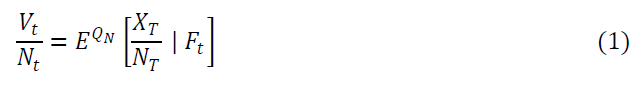or equivalently as: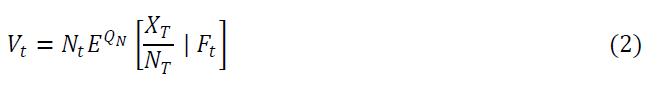where Vt is the option price evaluated at time t; Nt is the numeraire evaluated at time t; EQN is an expectation with respect to the measure of numeraire N; XT is at time T.

A European Option with payoff XT at time T takes the below form for a European Call: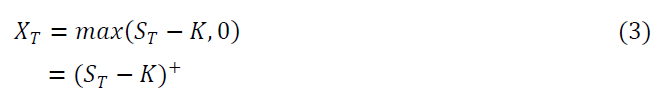and likewise for a European Put Option: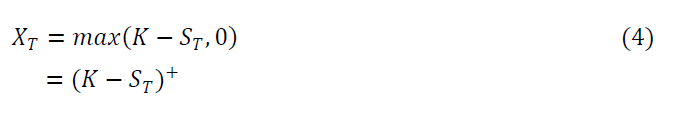3. ## Swap Present Value

An interest rate swaption is an option and an interest rate swap (IRS).In order to evaluate the swaption payoff we need to understand the IRS instrument and how to determine its price or present value.

In an interest rate swap transaction a series of fixed cashflows are exchanged for a series of floating cashflows. One may consder a swap as an agreement to exchange a fixed rate loan for a variable or floating rate loan. An extensive review of interest rate swaps, how to price and risk them is outlined in Burgess (2017a).

The net present value PV or price of an interest rate swap can be evaluated as follows.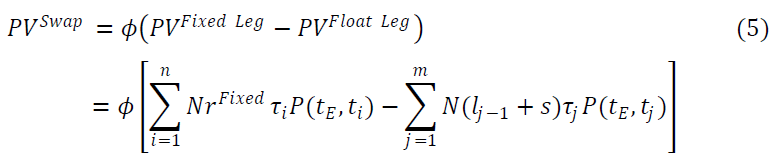where PVFixed Leg refers to The present value of fixed coupon swap payments. Receiver swaps receive the fixed coupons (and pay the floating coupons) and payer swaps pay the fixed coupons (and receive the floating coupons). The PVFloat Leg refers to the present value of variable or floating Libor coupon swap payments. Each coupon is determined by the Libor rate at the start of the coupon period. When the Libor rate is known the rate is said to have been fixed or reset and the corresponding coupon payment is known.

In the swaps market investors want to enter swaps transactions at zero cost. On the swap effective date or start date of the swap the swap has zero value, however as time progresses this will no longer be the case and the swap will become profitable or loss making. To this end investors want to know what fixed rate should be used to make the fixed and floating legs of a swap transaction equal, which we denote pMarket. Such a fixed rate is called the swap or par rate. Interest rate swaps are generally quoted and traded in the financial markets as par rates, i.e. the rate that matches the present value of the fixed leg PV and the float leg PV. Thus, swaps that are executed with the fixed rate being set to the par rate and called par swaps and they have a net PV of zero.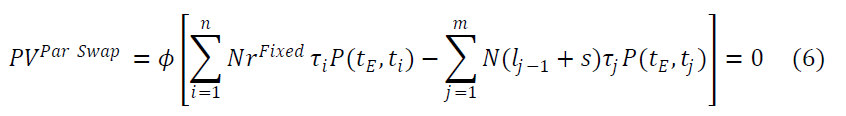Since par swaps have zero PV we derive,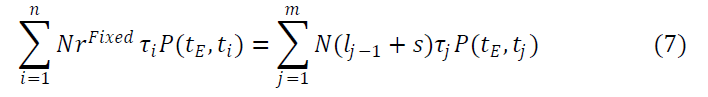Furthermore, par swaps have a fixed rate equal to the par rate, i.e. rFixed=pMarket.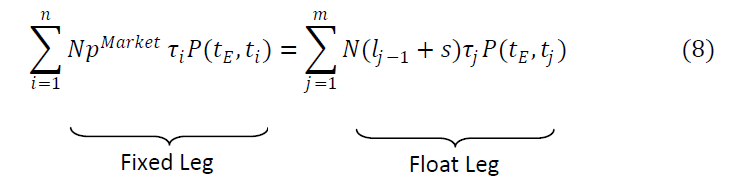Following Burgess (2017a) we can represent the float leg as a fixed leg traded at the market par rate pMarket and hence (8) becomes,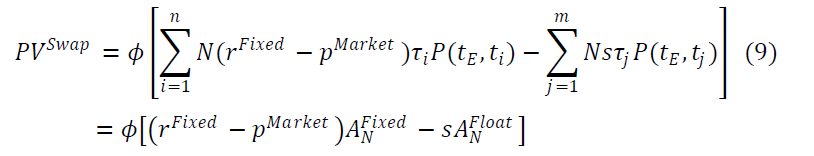In the case when there is no Libor spreads on the floating leg this simplifies to: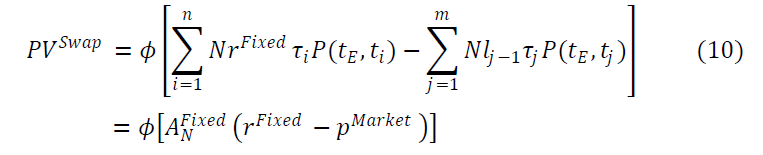4. ## Swaption Price

In a receiver swaption the holder has the right to receive the fixed leg cashflows in the underlying swap at a strike rate agreed today and pay the float leg cashflows. A rational option holder will only exercise the option if the fixed leg cashflows to be received are larger than the float leg cashflows to be paid. The corresponding option payoff XT can be represented as: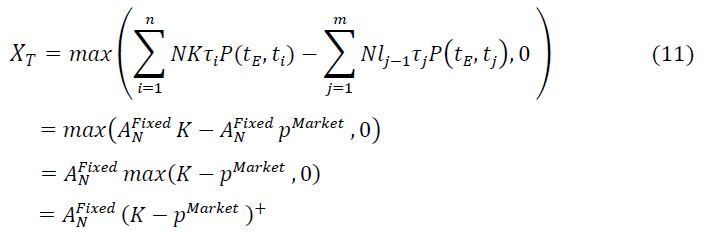As can be seen by comparing (11) and (4) a receiver swaption payoff replicates the payoff of a put option scaled by the swap fixed leg annuity AFixedN.

Likewise a payer swaption extends the holder the right to receive the fixed cashflows from the underlying swap and has payoff XT.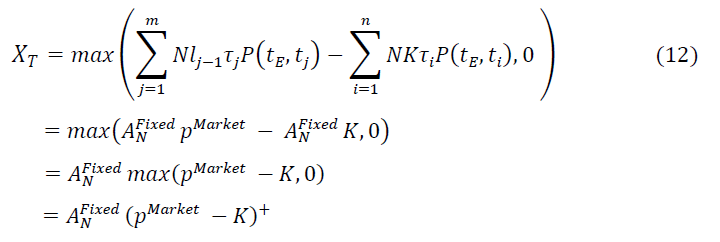Again by comparing (12) and (3) a payer swaption payoff replicates the payoff of a call option scaled by the swap fixed leg annuity AFixedN.

It can be easily seen from the swaption payoff that a payer swaption represents a call option payoff and a receiver swaption a put option payoff.

Both options give the right but not the obligation to enter into a swap contract in the future to pay or receive fixed cashflows respectively in exchange for floating cashflows with the fixed rate set today at the strike rate K.

In the general case we can represent a swaption payoff as,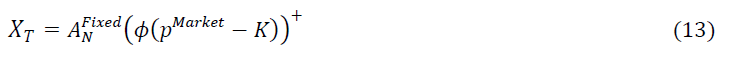where ϕ=1 for a payer swaption and -1 for a receiver swaption.

Applying the martingale representation theorem from section (2.1) we can price the swaption using equation (2) using the swaption payoff from (13) giving: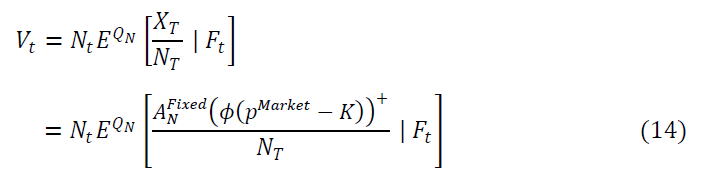Following Burgess (2017a) we may select a convenient numeraire to simplify the expectation term in (14). In this case we select the annuity measure AFixedN with corresponding probability measure QA which leads to,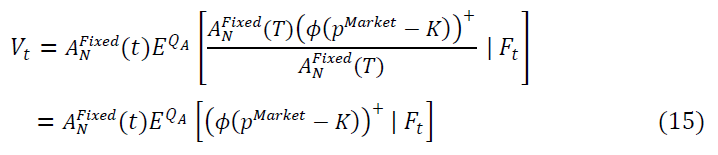We could at this stage see that expectation term in (15) can be evaluated using the generalized Black-Scholes (1973) formula as shown in (21) below. However for completeness we change the measure from the annuity measure QA to the more familiar and native Black-Scholes (1973) measure, namely the risk- neutral or savings account measure Q. This is merely to help readers identify the Black-Scholes expectation and is not an actual requirement.

Following Baxter (1966), Hull (2011) and Burgess (2014), we apply the Radon- Nikodym derivate allows us to change the numeraire and associated probability measure of an expectation and is often used in conjunction with the Martingale Respresentation Theorem. The Radon-Nikodym derivative (dQM/dQN) is defined as,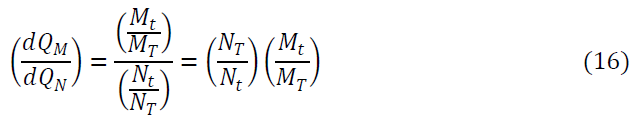To change numeraire from QN to QM, we can multiply Vt by Radon-Nikodym derivative (dQM/dQN) giving,Utilizing Radon-Nikodym derivative to change the measure from the annuity measure QA to the risk-neutral savings account measure Q in (15) leads to a generalized Black-Scholes formula type expression as shown below.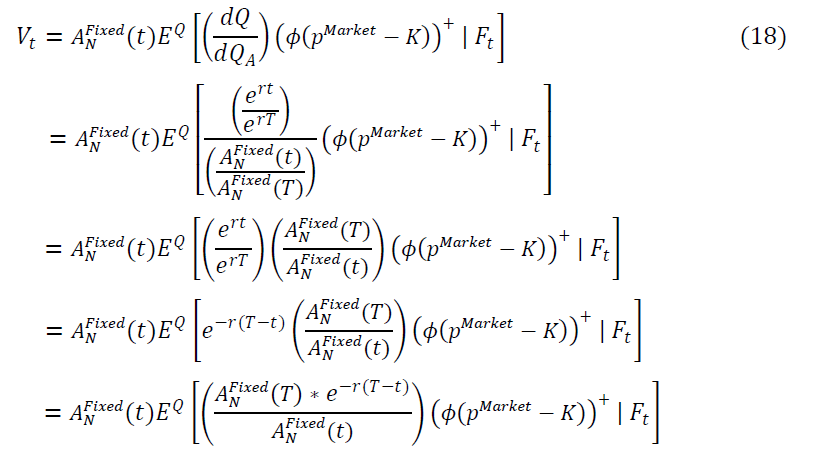Noting that e-r(T-t) is the discount factor operator from time T to t under savings account measure. If we discount the spot annuity AFixedN(T) back to time t by applying the discount factor operator we have the AFixedN(T)*e-r(T-t)=AFixedN(t) giving,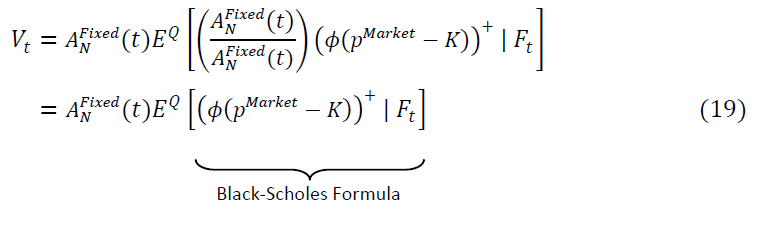In case where our underlying swap has a Libor spread on the floating leg using (9) gives,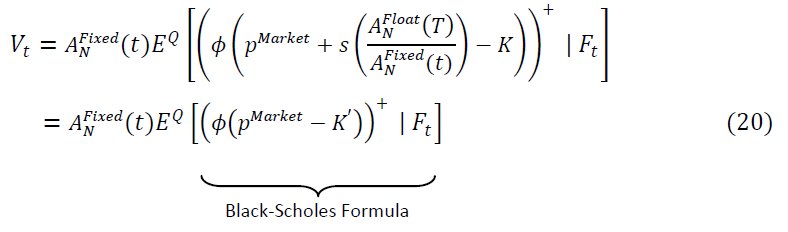where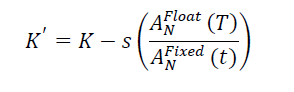5. ## Generalized Black-Scholes and Black-76 Formulae

The generalized Black-Scholes formula for European option pricing, see Black- Scholes (1973), is popular amongst traders and market practictions because of its analytical tractability. The formula relies heavily on dynamic delta hedging, see Derman and Taleb (2005) for details. It evaluates the price (Vt) at time t of a European option with expiry at time T as follows,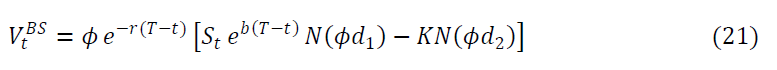where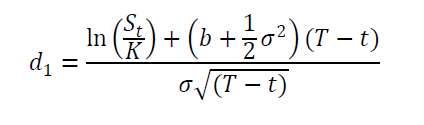and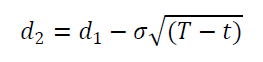Furthermore, as outlined in Burgess (2017b) setting the carry term b = 0 leads to the Black-76 formula for pricing interest rate options namely,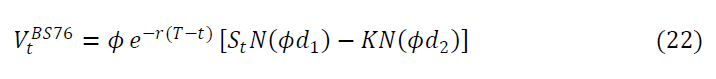whereandAs outlined in the appendix we should now recognise that the swaption pricing formula from (19) is nothing more than the generalized Black-Scholes (1973) formula scaled by the annuity factor AFixedN(t). In this particular case the underlying asset is an interest rate, therefore we customize the generalized Black- Scholes formula as outlined in Burgess (2017b) to price interest rate options by setting the carry term b to zero, which leads to the Black-76 formula, see Black (1976).

Note that comparing the Black-76 formula from (22) and our swaption pricing formula (19) we have additional discounting term e-r(T-t), which we eliminate by setting the zero rate r = 0 to make this additional term equal to unity.

Therefore, applying the generalized Black-Scholes (1973) result to (19) with the carry term b = 0 and zero rate r = 0 leads to following result. European swaptions can be priced using the Black-76 analytical formula scaled by the interest rate swap fixed leg annuity term AFixedN(t).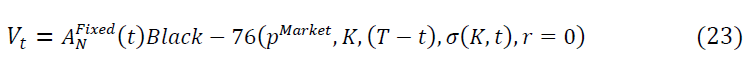quoting this explicitly we have,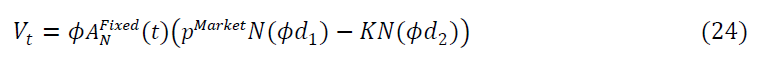where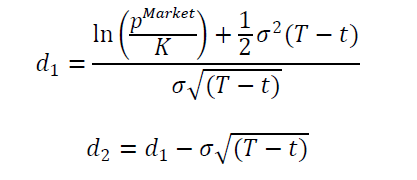and ϕ = 1 denotes a payer swaption and ϕ = -1 a receiver swaption. In the case where our underlying swap has a Libor floating spread we adjust the strike as outlined in (20) replacing K with K’ where6. ## Conclusion

In conclusion we reviewed the martingale representation theorem for pricing options, which allows us to price options under a numeraire of our choice. We also considered the classical European call and put option pricing payoffs to help us identify that payer swaptions are comparible to call options and likewise receiver swaptions to put options.

Since interest rate swaptions are options on interest rate swaps, we also discussed how to evaluate and price an interest swap to better understand the swaption payoff. In particular we highlight a key component of the underlying swap price is the annuity term, which was pivotal in selecting a numeraire to evaluate the expected swaption value.

We examined how to price interest rate swaptions using the Martingale Representation Theorem to derive a closed form analytical solution. We chose the annuity measure to simplify the expected swaption payoff. This reduced the pricing calculation to a Black-Scholes (1973) like expression. To make this more transparent we took an extra unnecessary step and applied the Radon-Nikodym derivative to change probability measure from the annuity measure to the savings account numeraire or risk-neutal measure, which is more classical and recongnizable, to arrive at a swaption pricing formula expressed in terms of the Black- 76 formula.

We showed that the interet swaption pricing formula is nothing more than the Black-76 formula scaled by the underlying swap annuity factor. In the appendix we also provide a full derivation of the generalized Black-Scholes formula for completeness.

## References

Baxter, M., and Rennie, A. (1966). Textbook: Financial Calculus – An Introduction to Derivatives Pricing. Cambridge University Press.

Black, F., and Scholes, M. (1973). The Pricing of Options and Corporate Liabilities. Journal of Political Economy, 81(3), 637-654.

Black, F. (1976). The Pricing of Commodity Contracts. Journal of Financial Economics, 3(1-2), 167-179.

Burgess, N. (2014). Martingale Measures & Change of Measure Explained. Available at SSRN: https://ssrn.com/abstract=2961006 or http://dx.doi.org/10.2139/ssrn.2961006

Burgess, N. (2017a). How to Price Swaps in Your Head - An Interest Rate Swap & Asset Swap Primer. Available at SSRN: https://ssrn.com/abstract=2815495 or http://dx.doi.org/10.2139/ssrn.2815495

Burgess, N. (2017b). A Review of the Generalized Black-Scholes Formula & It’s Application to Different Underlying Assets. Available at SSRN: https://ssrn.com/abstract=3023440 or http://dx.doi.org/10.2139/ssrn.3023440

Derman, E., and Taleb, N. (2005). The Illusion of Dynamic Delta Replication. Quantitative Finance, 5(4), 323-326.

Hull, J. (2011). Textbook: Options, Futures and Other Derivatives. 8th ed., Pearson Education Limited

## Appendix

A1. Derivation of the Generalized Black-Scholes Model

We first assume that the underlying asset St follows a Geometric Brownian Motion process with constant volatility σ namely,